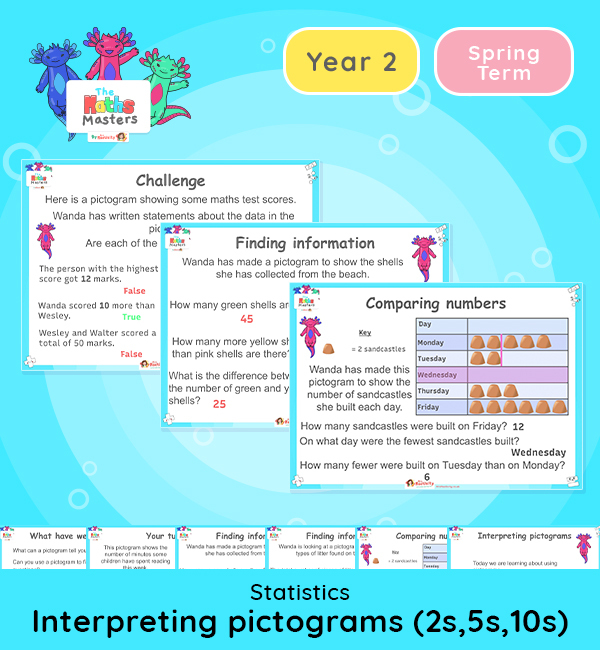Year 2 | Interpreting Pictograms (2, 5, 10) Lesson PresentationWhite Rose Year 2 statistics lesson plans

Aligned with the White Rose Maths scheme of work, this Year 2 | Interpreting Pictograms (2, 5, 10) Lesson Presentation is fully editable, and is designed for the Year 2 maths curriculum covering the following maths objectives for the autumn term:

Topic: Statistics

This lesson focuses on making and interpreting pictograms with 1 picture representing 2, 5 or 10 objects.

White Rose Maths Small Steps: Interpret pictograms (2,5, and 10).

NC Links: Interpret and construct simple pictograms, tally charts, block diagrams and simple tables. *Ask and answer simple questions by counting the number of objects in each category and sorting the categories by quantity. *Ask and answer questions about totalling and comparing categorical data.

TAF Statements: Greater Depth – Use reasoning about number and relationships to solve more complex problems and explain their thinking. *Solve unfamiliar word problems that involves more than one step.